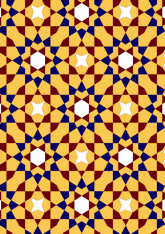data182/SYR0605
Revised: Graphic

## Geometry

• The symmetry group of the tiling is 2*22 (cmm).
• All the internal angles of the constituent polygons are a multiple of 7.5°.
• Contains two equilateral triangles.
• Contains one regular hexagon.
• Contains one regular four-pointed star polygon with vertex angle of 45°.
• There are 6 non-regular reflective tiles (including 2 kites).
• The tiling satisfies the two-colour condition.
• The tiling is edge-to-edge.
• As drawn, contains about 756 polygons.

## References

Publications referenced: